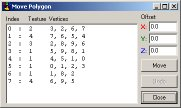• Rotate Vertices
• Use this function to rotate vertices AND associated lines and polygons.

• Axis to rotate around

• Specifies around which axis the vertices should be rotated. Check Show Axis in View Options Menu to see the axis symbol.

• Vertices to rotate

• Select if ALL vertices or only selected vertices should be rotated.
Note: Prior to this, select vertices and keep the Vertex Dialog open !

• Pivot point on axis

• Select whether the point of origin (0,0,0) or a specified vertex should be used as the pivot point.
If "Specified Vertex" is selected, a vertex has to be picked as pivot point.

Top of page

• Move Vertices
• Use this function to move vertices AND associated lines and polygons by certain values.

• Move all

• Moves ALL vertices with the given values.

• Move selected

• Select if ALL vertices or only selected vertices should be moved.
Note: Prior to this, select vertices and keep the Vertex Dialog open !

• Undo

• Undo restores the last move and can be used even after the Move dialog was closed and re-opened.

Top of page

• Mirror Vertices
• Use this function to mirror vertices around the point of origin.
Note: This function is not a "by plane" mirror. Values are mirrored around the point of origin, where X=100 will be X=-100 !

Associated Lines and Polygons are NOT mirrored !

• Keep Original

• Mirrors by the given values, but keeps the original vertices. This effectively doubles the mirrored vertices, increasing the total number of vertices.

• Mirror all

• Mirrors the given values of ALL vertices.

• Mirror selected

• Select if ALL vertices or only selected vertices should be mirrored.
Note: Prior to this, select vertices and keep the Vertex Dialog open !

• Undo

• Undo restores the last mirror and can be used even after the Mirror dialog was closed and re-opened.

Top of page

• Scale Vertices
• Use this function to scale vertices AND associated Lines and Polygons around the point of origin.

Associated Lines and Polygons are also scaled !

• X,Y,Z

• Only the specified values are scaled. This causes a distortion in the specified axis.
Example: Lower the total height of a house, but keep the foot-print the same.

• All Directions

• Scales the objects equally to all sides.

• Scale all

• Scales the given values of ALL vertices.

• Scale selected

• Select if ALL vertices or only selected vertices should be scaled.
Note: Prior to this, select vertices and keep the Vertex Dialog open !

• Undo

• Undo restores the last scale and can be used even after the Scale dialog was closed and re-opened.

Top of page

• Center All Vertices
• Use this function to center all vertices AND associated Lines and Polygons around the point of origin.

• Undo

• Undo restores the last center and can be used even after the Center dialog was closed and re-opened.

Top of page

• Copy Polygons
• Use this function to copy polygons AND associated vertices and Lines from one point to another.
Select one or more polygons from the list before using the function.

• Offset

• Specifiy the values in X,Y,Z by which the polygon(s) should be copied.

• Visible from:

• - Opposite Side:
On the destination polygon(s), makes textures appear from the opposite side.

Example: A banner across the track. Make one textured polygon, then copy it by a small value, which will make the banner two-sided.

- Same Side:
On the destination polygon(s), makes textures appear from the same side as the source polygon(s). In this case, all polygons face the same direction.

• Texture Orientation:

• - Same Orientation:
On the destination polygon(s), makes textures appear the same as on the source polygon(s).

- Mirrored:
On the destination polygon(s), makes textures mirrored vertically.

- Untextured:
Destination polygon(s) get no texture.

• Undo

• Undo restores the last copy and can be used even after the Copy Polygon dialog was closed and re-opened.

Top of page

• Move Polygons
• Use this function to move Polygons AND associated vertices and lines by certain values.
Select one or more polygons from the list before using the function.
Note: The result of this function is is basically the same as with Move Vertices.
However, once polygons are drawn, it's much easier to use.• Offset

• Specifiy the values in X,Y,Z by which the polygon(s) should be moved.

• Move

• Moves selected polygons with the given values.

• Undo

• Undo restores the last move and can be used even after the Move dialog was closed and re-opened.

Top of page